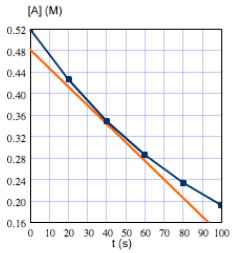# Problem: For the reactionA → productstime and concentration data were collected.t(s)    [A](M)0       0.5220     0.4340     0.3560     0.2980     0.24100   0.20The blue curve is the plot of the data. The orange line is tangent to the blue curve at t = 40 s.Approximate the instantaneous rate of this reaction at time t = 40 s. ____ M/s

###### FREE Expert Solution###### Problem Details

For the reaction

A → products

time and concentration data were collected.

t(s)    [A](M)

0       0.52

20     0.43

40     0.35

60     0.29

80     0.24

100   0.20

The blue curve is the plot of the data. The orange line is tangent to the blue curve at t = 40 s.Approximate the instantaneous rate of this reaction at time t = 40 s.

____ M/s